Test: Dimensional & Model Analysis - 1

# Test: Dimensional & Model Analysis - 1 - Civil Engineering (CE)

Test Description

## 10 Questions MCQ Test GATE Civil Engineering (CE) 2024 Mock Test Series - Test: Dimensional & Model Analysis - 1

Test: Dimensional & Model Analysis - 1 for Civil Engineering (CE) 2023 is part of GATE Civil Engineering (CE) 2024 Mock Test Series preparation. The Test: Dimensional & Model Analysis - 1 questions and answers have been prepared according to the Civil Engineering (CE) exam syllabus.The Test: Dimensional & Model Analysis - 1 MCQs are made for Civil Engineering (CE) 2023 Exam. Find important definitions, questions, notes, meanings, examples, exercises, MCQs and online tests for Test: Dimensional & Model Analysis - 1 below.
Solutions of Test: Dimensional & Model Analysis - 1 questions in English are available as part of our GATE Civil Engineering (CE) 2024 Mock Test Series for Civil Engineering (CE) & Test: Dimensional & Model Analysis - 1 solutions in Hindi for GATE Civil Engineering (CE) 2024 Mock Test Series course. Download more important topics, notes, lectures and mock test series for Civil Engineering (CE) Exam by signing up for free. Attempt Test: Dimensional & Model Analysis - 1 | 10 questions in 30 minutes | Mock test for Civil Engineering (CE) preparation | Free important questions MCQ to study GATE Civil Engineering (CE) 2024 Mock Test Series for Civil Engineering (CE) Exam | Download free PDF with solutions
 1 Crore+ students have signed up on EduRev. Have you?
Test: Dimensional & Model Analysis - 1 - Question 1

### Euler number or pressure coefficient may be represented as (notations have their usual meanings)

Test: Dimensional & Model Analysis - 1 - Question 2

### A spillway of an irrigation project is to be studied by means of a model constructed to a scale of 1 : 9. The prototype discharge is 1000 m3/s. Neglecting the viscous and surface tension effects, the required flow rate for the model is

Detailed Solution for Test: Dimensional & Model Analysis - 1 - Question 2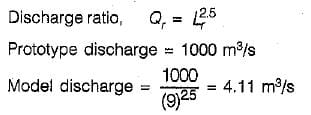Test: Dimensional & Model Analysis - 1 - Question 3

### Match List-I (Equation/Principie) with List-ll (Property) and select the correct answer using. the codes given below the lists: List-I A. Energy equation B. Continuity equation C. Moment of momentum principle D: Impulse-momentum principle List-ll 1. Force on a moving vane 2. Lawn sprinkler 3. Pressure at a point in a pipeline 4. Flow at two sections of a tapering pipe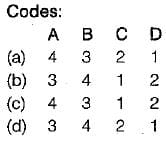Test: Dimensional & Model Analysis - 1 - Question 4

The distorted models, used in the hydraulic studies, are those which

Detailed Solution for Test: Dimensional & Model Analysis - 1 - Question 4

Distorted - model can have vertical scale exaggeration or slope exaggeration. Therefore they are not geometrically similar. It may have either geometrical distortion, or material distortion or distortion of hydraulic quantities or a combination of these.

Test: Dimensional & Model Analysis - 1 - Question 5

A 1: 30 scale model of a submarine is to be tested in a wind tunnel for its drag when it is operating at 15 km/h in ocean. The kinematic viscosity of air is 1.51 x 10-5 m2/s and for water 1.02 x 10-6 m2/s. What is the velocity of air in wind tunnel that should be maintained for kinematic similarity?

Detailed Solution for Test: Dimensional & Model Analysis - 1 - Question 5

The Reynold’s model law shall be applied in the case of fully submerged objects,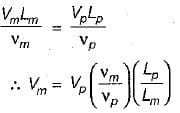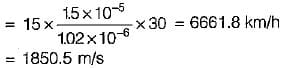The Reynold’s model law ensures dynamic; kinematic and geometric similarities.

Test: Dimensional & Model Analysis - 1 - Question 6

In model similarity, if gravitational and inertial forces are the only important forces, then what is the discharge ratio?
where Lr = ratio of length dimension.

Detailed Solution for Test: Dimensional & Model Analysis - 1 - Question 6

If gravitational and inertial forces are the only important forces, then the Froude number must be the same in the model and prototype. Thus,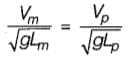But g is same for both model and prototype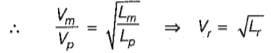Now, Discharge = Area x Velocity
⇒ Qr = Ar x Vr ⇒ Qr = Lr2 x (Lr)1/2
⇒ Qr = Lr5/2

Test: Dimensional & Model Analysis - 1 - Question 7

Which ratio is the Reynold's Number?

Detailed Solution for Test: Dimensional & Model Analysis - 1 - Question 7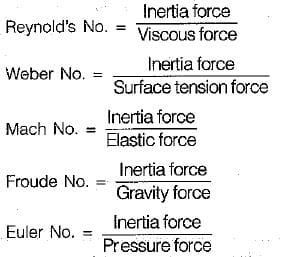Test: Dimensional & Model Analysis - 1 - Question 8

An orifice is discharging under a head of 1.25 m of water. A Pitot tube kept at its centre line at the vena contracta indicates a heap of 1.20 m of water. The coefficient of velocity of the orifice is

Detailed Solution for Test: Dimensional & Model Analysis - 1 - Question 8

h0 be the head of Orifice
hp be the head of Pitot tube
Velocity of emergence of water from orifice can be given by  =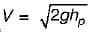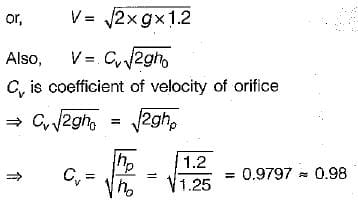Test: Dimensional & Model Analysis - 1 - Question 9

The geometrical similarity between model and prototype is achieved by

Detailed Solution for Test: Dimensional & Model Analysis - 1 - Question 9

Geometric similarity exists between the model and the prototype if the ratios of corresponding length dimensions in the model and the prototype are equal. Different model scales for horizontal and vertical dimension are used in a distorted model. Geometric similarity is achieved by using same model scale throughout.

Test: Dimensional & Model Analysis - 1 - Question 10

Froude number is the ratio of inertia force to

## GATE Civil Engineering (CE) 2024 Mock Test Series

28 docs|296 tests
Information about Test: Dimensional & Model Analysis - 1 Page
In this test you can find the Exam questions for Test: Dimensional & Model Analysis - 1 solved & explained in the simplest way possible. Besides giving Questions and answers for Test: Dimensional & Model Analysis - 1, EduRev gives you an ample number of Online tests for practice

## GATE Civil Engineering (CE) 2024 Mock Test Series

28 docs|296 tests